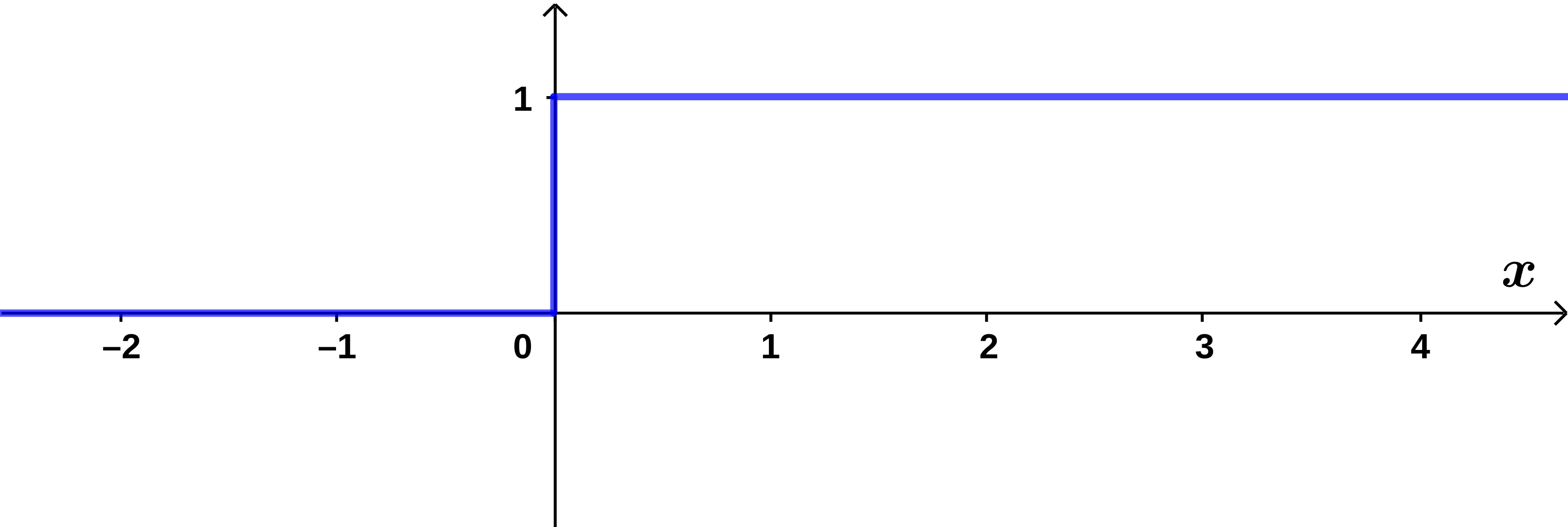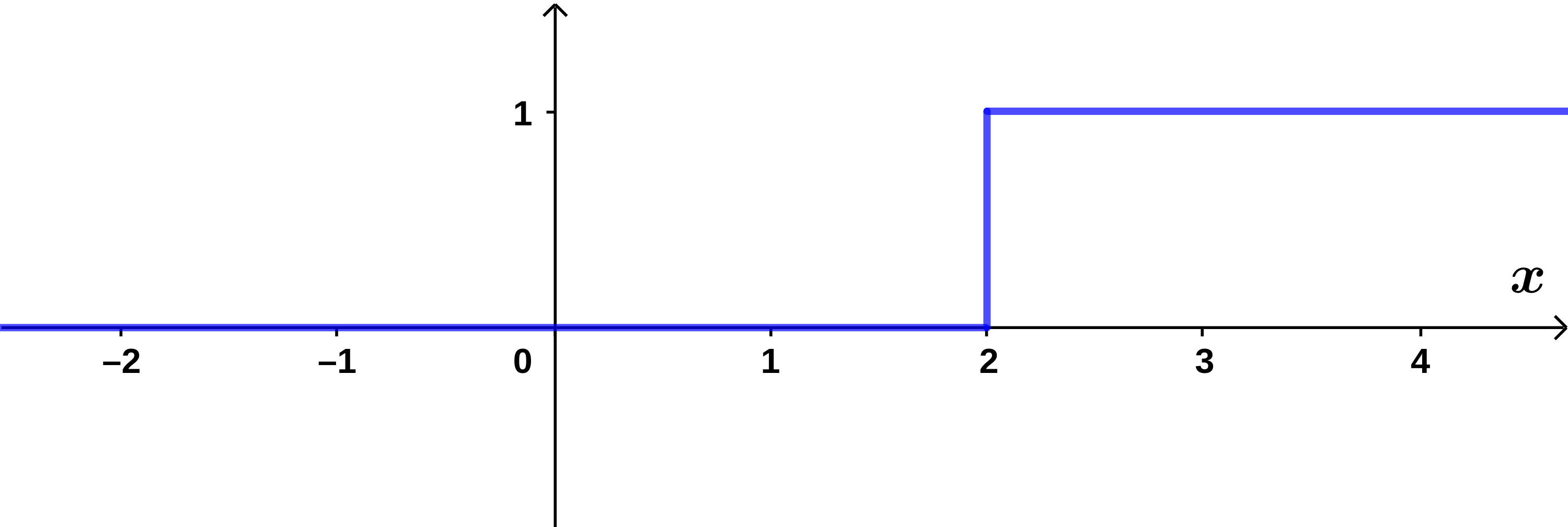## Section11.1Step Functions

The step function $$\Theta(x)\text{,}$$ also called the Heaviside function or theta function, is defined to be $$0$$ if $$x\lt 0$$ and $$1$$ if $$x>0\text{.}$$ See Figure 11.1.1.Figure 11.1.1. The step function $$\Theta(x)\text{.}$$

Step functions are used to model idealized physical situations where some quantity changes rapidly from one value to another in such a way that the exact details of the change are irrelevant for the solution of the problem, e.g. edges of materials or a process that switches on abruptly at a particular time, etc.

By shifting the argument of the function, it is possible to put the discontinuity of the theta function wherever we need it. In Figure 11.1.2 you can see that the graph of $$\Theta(x-2)$$ has discontinuity at $$x=2\text{,}$$ instead of at $$x=0\text{.}$$Figure 11.1.2. The function $$\Theta (x-2)\text{.}$$

The the step function can also be used to turn a function off, since

\begin{equation} \Theta(-x) = 1-\Theta(x)\tag{11.1.1} \end{equation}

is a step down, rather than up.

Make sure that you are able to graph all of the following functions:

\begin{align*} f(x) \amp = 2\Theta(x) ,\\ f(x) \amp = \Theta(x-2) ,\\ f(x) \amp = \Theta(2x-3) ,\\ f(x) \amp = \Theta(2-x) ,\\ f(x) \amp = \Theta(x)\> \Theta(x-2) ,\\ f(x) \amp = \Theta(x)-\Theta(x-2) ,\\ f(x) \amp = \sin x\> \Theta(x-\frac{\pi}{2}) . \end{align*}

In most physical problems, it is unnecessary to know the exact value of $$\Theta(x)$$ at the discontinuity. If it ever matters, it is usually easiest to define it symmetrically, i.e.

\begin{equation} \Theta(0) = \frac12 .\tag{11.1.2} \end{equation}